# AP Chemistry : VSEPR Theory

## Example Questions

### Example Question #1 : Vsepr Theory

Find the bond angle present in sulfur dioxide.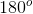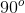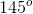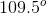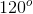Explanation:

Sulfur dioxide has the formula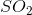and takes on a trigonal planar electronic geometry, with the two oxygen atoms and the lone pair in the same plane. The molecular geometry will be bent, resulting in a oxygen-sulfur-oxygen bond angle of 120 degrees.

### Example Question #1 : Vsepr Theory

Which answer option best approximates the bond angle present in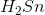?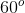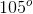Explanation:

This is the Lewis structure of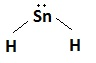Since the tin atom (Sn) is bonded to two atoms and has one lone pair, the molecule is sp2 hybridized and has a bent, or angular shape. Bent molecules have bond angles of approximately 120°.

### Example Question #2 : Vsepr Theory

Which term best describes the molecular geometry of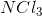?

Trigonal planar

Bent

Trigonal pyramidal

Trigonal bipyramid

Tetrahedral

Trigonal pyramidal

Explanation:

This is the Lewis structure of.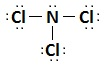has three bound atoms and one lone pair, so it is sp3 hybridized and trigonal pyramidal. Note that the electronic geometry (geometry including the lone pair) is tetrahedral, but the molecular geometry (excluding the lone pair) is trigonal pyramidal.

### Example Question #4 : Vsepr Theory

Which of the following ions is planar?

All of these are planar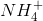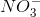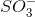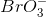Explanation:

This Lewis structure is for nitrate,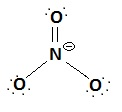The nitrogen atom is bonded to three other atoms and doesn't have any lone pair electrons, so this atom will be sp2 hybridized. The shape of any sp2 hybridized molecule, without lone pairs, is trigonal planar. None of the other ions are both sphybridized and without lone pairs.

### Example Question #5 : Vsepr Theory

What will be the molecular shape of a molecule consisting of a central atom surrounded by two bonding pair electrons and two electron lone pairs?

Trigonal pyramidal

Bent

Trigonal bipyramid

Linear

Tetrahedral

Bent

Explanation:

A molecule consisting of a central atom surrounded by two bonding pair electrons and two lone pairs will always be sp3 hybridized. The molecular shape of an sp3 hybridized molecule with two lone pairs is bent/angular. Here is an example of a sp3 hybridized molecule with two lone pairs.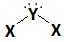Note that the electronic geometry for this molecule will be tetrahedral, if the lone pairs are included in the geometry.

### Example Question #6 : Vsepr Theory

According to VSEPR theory, what will be the shape of the following molecule: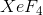Square planar

See-saw

Octahedral

Square pyramidal

Tetrahedral

Square planar

Explanation:

The basic principle of VSEPR theory is that atoms bonded to a central atom will all push each other as far away from each other as they can be. They will be pushed even harder by lone pairs. To understand what kind of shape is going to be present in a certain molecule, we need to understand how many bonding atoms there are and how many lone pairs there are on the central atom.

The central atom in this case is xenon (Xe), which we can tell primarily because it is the least electronegative. Xenon has 8 valence electrons, and fluorine has 7 valence electrons. Each of the 4 fluorine atoms needs to borrow one electron from xenon to form the covalent bond. This leaves xenon with 4 bonds and 4 leftover valence electrons, or two lone pairs.

The moelcule's steric number is 4 bonds + 2 lone pairs = 6. We want to think of a shape that has 6 equally spaced corners; usually this is called "octahedral." Think of two square-based pyramids being pressed together, base-to-base. This shape will result from any central atom with six substituents.

However, for our molecule, since two "substituents" are lone pairs and not actual atoms, they are not considered a part of the shape of the molecule, though they still affect the other four bonded atoms. To reduce strain and promote molecular symmetry, the two lone pairs are distanced from each other and the four atoms exist in a single plain, resulting in a square planar geometry.

According to VSEPR, molecules with 4 bonding atoms and 2 lone pairs, will have the square planar shape.

### Example Question #7 : Vsepr Theory

Which of the following molecules has a central atom with a trigonal planar configuration?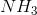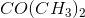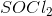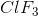Explanation:

For this question, we're asked to identify an answer choice that represents a central atom with a trigonal planar shape.

When looking at each compound, the first thing we need to do is determine the central atom. Generally, this atom will have the lowest electronegativity. Then, we need to determine the number of valence electrons the central atom has. We do the same thing for all the atoms attached to the central atom, and then we draw a bond between the central atom and each connecting atom. Next, we need to check to see if the central atom has at least a full octet of eight electrons (although, elements in the third period and below can go beyond this due to their d orbitals). We also check to see if the connecting atoms have a full octet. If not, then we can either draw additional bonds between the central atom and the connecting atom(s). If the central atom cannot contribute anymore bonds, however, we'll need to add additional electrons to fill the octet of each connecting atom, which would then give us a polyatomic ion. With this set of rules in mind, let's take a look at each answer choice and evaluate the shape of its central atom.

Let's take a look at. In this compound, the central atom is nitrogen, which has five valence electrons. To fill its octet, nitrogen needs to gain three more electrons. In this compound, it does this by forming three single bonds with other atoms (in this case, three hydrogen atoms). Also, since each hydrogen atom now contains two electrons (one from itself, and the other from nitrogen), the hydrogen is stable because it will only go up to two electrons. The nitrogen is also stable. It's important to note that the nitrogen in this case also has a lone pair of electrons. As a result, ammonia will have a pyrimidal conformation.

Next, let's look at. In this case, chlorine is the central atom. After forming a single bond with each of three florine atoms, all of the atoms will be stable. The chlorine atom will have three single bonds and two different lone pairs of electrons. As a result, this compound will have a T-shaped geometry.

The central atom foris sulfur. In order to make all the atoms happy in this compound, sulfur will form a double bond with the oxygen and a single bond to each of the chlorine atoms. As a result, the central sulfur atom will have three total atoms to which it's bound, as well as one lone electron pair. Thus, this compound will have a pyrimidal geometry.

Lastly, let's look at. To stabilize all the atoms, the central carbon atom will have a double bond with the oxygen and two single bonds, one to each methyl group. Consequently, this molecule's central carbon atom is bound to a total of three atoms, and it has no lone electron pairs. As a result, this compound will adopt a trigonal planar geometry.

### All AP Chemistry Resources